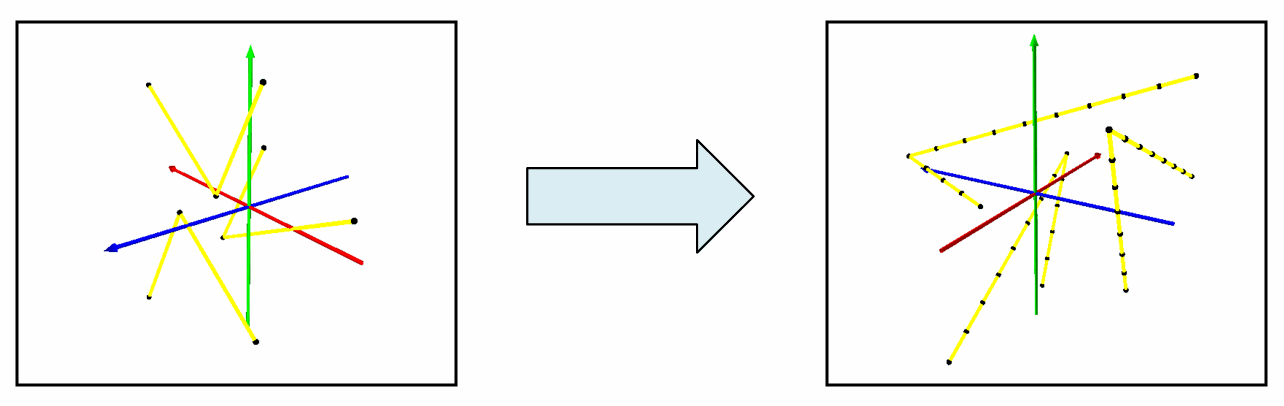This document is archived and information here might be outdated.  Recommended version.

IPolycurve3D.Densify3D Method (ArcObjects .NET 10.7 SDK)
 ArcObjects Help for .NET developers > ArcObjects Help for .NET developers > ArcObjects namespaces > Geometry > ESRI.ArcGIS.Geometry > Interfaces > IP > IPolycurve3D Interface > IPolycurve3D.Densify3D Method
 ArcGIS Developer Help

# IPolycurve3D.Densify3D Method

Converts this Polycurve into a piecewise linear approximation of itself.

```[Visual Basic .NET]
Public Sub Densify3D ( _
ByVal maxSegmentLength As Double, _
ByVal maxDeviation As Double _
)
```
```[C#]
public void Densify3D (
double maxSegmentLength,
double maxDeviation
);
```
```[C++]
HRESULT Densify3D(
double maxSegmentLength,
double maxDeviation
);
```
```[C++]
Parameters
maxSegmentLength
maxSegmentLength is a parameter of type double
maxDeviation
maxDeviation is a parameter of type double
```

#### Product Availability

Available with ArcGIS Engine, ArcGIS Desktop, and ArcGIS Server.

#### Description

Converts Polycurve into a Polycurve composed of Line segments with 3D length maxSegmentLength (or less) that are within maxDeviation of the original polycurve.  If maxDeviation = 0, maxDeviation is ignored and Line segments with length maxSegmentLength are constructed with vertices laying on the original curve.  All of the segments in the final polyline will be 3D Lines.

[C#]public static void Densify3D()

{

const double DensifyMaxSegmentLength = 2.43;

const double DensifyMaxDeviation = 2;

IGeometry polylineGeometry = GetPolylineGeometry();

IPolycurve3D polycurve3D = polylineGeometry as IPolycurve3D;

polycurve3D.Densify3D(DensifyMaxSegmentLength, DensifyMaxDeviation);

}